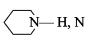$PC{l}_{5}$ exists but $NC{l}_{5}$ does not because:

(a) nitrogen has no vacant 2d-orbitals

(b)$NC{l}_{5}$ is unstable

(c) nitrogen atom is much smaller than p

(d) nitrogen is highly inert

Concept Questions :-

Acid, Base and Fajan's Rule
High Yielding Test Series + Question Bank - NEET 2020

Difficulty Level:

Bond angle between two hybrid orbitals is ${105}^{°}$.% S character orbital is:

1. between 30-31%

2. between 19-20%

3. between 25 -26%

4. between 22-23%

Concept Questions :-

Hybridisation
High Yielding Test Series + Question Bank - NEET 2020

Difficulty Level:

Which bond angle would result in the maximum dipole moment for the triatomic molecule $X{Y}_{2}$ shown below?

(a) ${90}^{°}$

(b) ${120}^{°}$

(c) ${150}^{°}$

(d) ${180}^{°}$

Concept Questions :-

Polarity
High Yielding Test Series + Question Bank - NEET 2020

Difficulty Level:

The hydrogen bonding is strongest in:

(a) $O—H‐‐‐S$

(b) $S—H‐‐‐O$

(c) $F—H‐‐‐F$

(d) $F—H‐‐‐O$

Concept Questions :-

Hydrogen Bonding
High Yielding Test Series + Question Bank - NEET 2020

Difficulty Level:

The ionisation energy will be maximum for the process:

(a) $Ba\to B{a}^{2+}$

(b) $Be\to B{e}^{2+}$

(c) $Cs\to C{s}^{+}$

(d) $Li\to L{i}^{+}$

Concept Questions :-

Ionic Bonding
High Yielding Test Series + Question Bank - NEET 2020

Difficulty Level:

In which of the process, the bond order increases and magnetic behaviour changes?

(a) ${N}_{2}\to {{N}_{2}}^{+}$

(b) ${C}_{2}\to {{C}_{2}}^{+}$

(c) $NO\to N{O}^{+}$

(d) ${O}_{2}\to {{O}_{2}}^{+}$

Concept Questions :-

M.O.T
High Yielding Test Series + Question Bank - NEET 2020

Difficulty Level:

In piperidineatom has hybridization:

1. sp

2. $s{p}^{2}$

3. $s{p}^{3}$

4. $ds{p}^{2}$

Concept Questions :-

Hybridisation
High Yielding Test Series + Question Bank - NEET 2020

Difficulty Level:

Specify the coordination geometry around and hybridization of N and B atoms in a 1:1 complex of $B{F}_{3}$ and $N{H}_{3}$:

(a) N: tetrahedral, $s{p}^{3}$; B : tetrahedral, $s{p}^{3}$

(b) N: pyramidal, $s{p}^{3}$; B : pyramidal, $s{p}^{3}$

(c) N: pyramidal, $s{p}^{3}$; B: planar, $s{p}^{2}$

(d) N: pyramidal, $s{p}^{3}$; B: tetrahedral, $s{p}^{3}$

High Yielding Test Series + Question Bank - NEET 2020

Difficulty Level:

Assuming $2s-2p$ mixing is NOT operative, the paramagnetic species among the following is :

(a) $B{e}_{2}$

(b) ${B}_{2}$

(c) ${C}_{2}$

(d) ${N}_{2}$

High Yielding Test Series + Question Bank - NEET 2020

Difficulty Level:

When ${O}_{2}$ is adsorbed on a metallic surface, electron transfer occurs from the ,metal to ${O}_{2}$. The wrong statements(s) regarding this adsorption is (are):

(a) ${O}_{2}$ is physisorbed

(b) Heat is released

(c) Occupancy of $\pi *2p$ of ${O}_{2}$ is increased

(d) Bond length of ${O}_{2}$ is increased

Concept Questions :-

Covalent Bonding